• 第一节首先引入拓扑空间 0.前置知识（ 幂集、子集族和连续的开集刻画） 0.1 幂集和子集族 设XXX为非空集合，记2X2^X2X是XXX的全体子集（包含XXX及空集∅\varnothing∅）的集合，称为XXX的幂集。把2X2^X2X的子集（即...
没错，这个系列是我无法安心复习而使用记博客的方式来对拓扑学知识重点（考点）进行汇总总结。以尤承业老师的《基础拓扑学讲义》为基础。
第一节首先引入拓扑空间、度量空间等基础概念。
文章目录0.前置知识（幂集和子集族）幂集和子集族1.拓扑空间1.1 拓扑空间定义1.2 闭集1.3 “特殊”的拓扑2.度量拓扑2.1 度量空间的定义2.2 度量拓扑3.子空间4.拓扑空间的几个基本概念4.1 邻域、内点与内部4.2 聚点与闭包4.3 稠密与可分拓扑空间4.4 序列收敛
0.前置知识（幂集和子集族）
幂集和子集族
设$X$为非空集合，记$2^X$是$X$的全体子集（包含$X$及空集$\varnothing$）的集合，称为$X$的幂集。把$2^X$的子集（即以$X$的一部分子集为成员的集合）称为$X$的子集族。
1.拓扑空间
1.1 拓扑空间定义
设$X$为一非空集合，$X$的一个子集族$\tau$称为$X$的一个拓扑，如果它满足下列三条拓扑公理：
（1）$X，\varnothing$都包含在$\tau$中。
（2）$\tau$中任意个成员的并集仍在$\tau$中。
（3）$\tau$中有限个成员的交集仍在$\tau$中。
（$3'$)（3）的等价表述：$\tau$中两个成员的交集仍在$\tau$中。
集合$X$和它的一个拓扑$\tau$一起称为一个拓扑空间，记作$(X,\tau)$，称$\tau$中的成员为这个拓扑空间的开集。
所以拓扑的实质是$X$的一个满足拓扑公理的子集族。给出集合的一个拓扑，就是规定它的哪些子集是开集。这种规定不是任意的，其必须满足三条拓扑公理。但一般来说，一个集合上可以规定许多不同的拓扑，因此说到一个拓扑空间时，要同时指明集合及所规定的拓扑，也常常只用集合来称呼一个拓扑空间，如拓扑空间$X$等。
即然有了开集，那我们是是不是要说一下 “闭集” 酱呢。
1.2 闭集
（1）闭集的定义： 拓扑空间$X$的一个子集A称为闭集，如果$A^c$是开集。（开集的余集）
eg:  $X$的离散拓扑$2^X$，任何子集都是开集，从而任何子集也都是闭集。
（2）闭集的3个重要性质：
one：$X$与$\varnothing$都是闭集。
因为$\varnothing,X$必定为开集，而她们的补集又是彼此，所以对于任何拓扑空间$\varnothing,X$必定为闭集。
two：任意多个闭集的交集是闭集。
three：有限多个闭集的并集是闭集。
为说明two和Three，这里先介绍一个重要的集合运算定律：摩根定律（De Morgan公式）
$B\backslash\bigcup_{\lambda \in \Lambda}A_\lambda = \bigcap_{\lambda \in \Lambda}(B\backslash A_\lambda),\tag{1}$
$B\backslash\bigcap_{\lambda \in \Lambda}A_\lambda = \bigcup_{\lambda \in \Lambda}(B\backslash A_\lambda) \tag{2}$
把$B$换称全集，$(B\backslash A_\lambda)=（A_\lambda）^c$
这个摩根定律在拓扑，概率论，实变函数都常常用到，需要牢记。
所以由摩根定律和拓扑公理(2)(3)知，two和three成立。
1.3 “特殊”的拓扑
“最大”与“最小”的拓扑。
（1）离散拓扑：设$X$为一非空集合，显然$X$的幂集$2^X$构成$X$的拓扑$\tau_s$，称为$X$上的离散拓扑。
显然$X$的任意拓扑$\tau \subset \tau_s$。
（2）平凡拓扑：{$X,\varnothing$}也是$X$上的拓扑，称为$X$上的平凡拓扑$\tau_t$。
同样，对于$X$的任意拓扑$\tau$，有$\tau_t \subset \tau$。
"大白话”拓扑
（3）余有限拓扑：设$X$是无穷集合，$\tau_f$={$A^c$ | $A$是$X$的有限子集}$\bigcup${$\varnothing$}，不难验证$\tau_f$是$X$的一个拓扑，称为$X$上的余有限拓扑。
（4）余可数拓扑：设$X$是无穷集合，$\tau_c$={$A^c$ | $A$是$X$的可数子集}$\bigcup${$\varnothing$}，不难验证$\tau_c$是$X$的一个拓扑，称为$X$上的余可数拓扑。
（5）欧式拓扑：设R是全体实数的集合，规定$\tau_e$={$U$ | $U$是若个开区间的并集}，这里 “若干个” 可以是无穷，有限，也可以是0，因此$\varnothing\in \tau_e$，进一步可以验证$\tau_e$是R上的拓扑，称为R上的欧式拓扑，记为$E^1=(R,\tau_e)$。
（记住这个”若干个“,😁)
既然都叫拓扑“空间"了，那怎么能少了“度量”呢（叉腰）
2.度量拓扑
2.1 度量空间的定义
集合$X$的一个度量$d$是一个映射，$d:X\times X\rightarrow R$,它满足：
（1）正定性：$d(x,x)=0，\forall x\in X \\d(x,y)>0$，当$x\not=y$时；
（2）对称性：$d(x,y)=d(y,x),\forall x,y\in X$；
（3）三角不等式：
$d(x,z)\leq d(x,y)+d(y,z)，\forall x,y,z\in X$
当集合$X$规定一个度量$d$后，称为度量空间，记作$(X,d)$。
eg：n维欧式空间
记$R^n=${$(x_1,x_2,...,x_n) | x_i\in R,i=1,...,n$}。规定$R^n$上的度量$d$为：
$d((x_1,x_2,...,x_n),(y_1,y_2,...,y_n))=\sqrt{\sum_{i=1}^n(x_i-y_i)^2},$
不难验证$d$满足正定性、对称性和三角不等式。记$E^n=(R^n,d)$，称为n维欧氏空间。
那么有了度量空间，怎样生成一个拓扑呢？或者说“度量拓扑”到底是什么呢？
2.2 度量拓扑
设$(X,d)$是一个度量空间，下面规定$X$的一个拓扑。
首先介绍球形域的概念，这是我们寻找拓扑的基础。
（1）球形领域：设$x_0\in X,\epsilon$是一正数，称$X$的子集：
$B(x_0,\epsilon):=\lbrace x\in X| d(x_0,x)<\epsilon \rbrace$
为以$x_0$为球心，$\epsilon$为半径的球形邻域。
（2）引理：$(X,d)$的任意两个球形领域的交集是若干个球形领域的并集。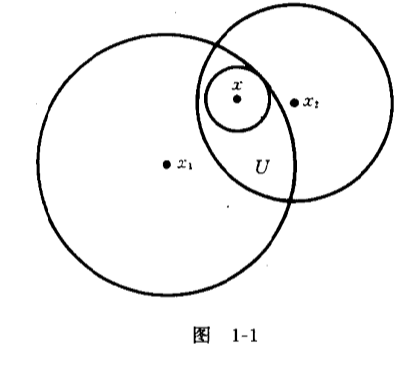证明：设$U=B(x_1,\epsilon_1) \bigcap B(x_2,\epsilon_2)$,$\forall x\in U$,则$\epsilon_i-d(x,x_i)>0(i=1,2)$（看图1-1）。记$\epsilon_x=min\lbrace \epsilon_1-d(x,x_1),\epsilon_2-d(x,x_2) \rbrace$，不难验证$B(x,\epsilon_x) \subset U$，于是$U=\bigcup_{x \in U}B(x,\epsilon_x).$
规定$X$的子集族$\tau_d=\lbrace U| U$是若干个球形领域的并集$\rbrace$
命题：实际上$\tau_d$就是我们要的度量空间的一个拓扑。
证明：
验证拓扑公理(1）
因为：0个球形邻域的并集即$\varnothing$（"若干个”😀），所以$\varnothing \in \tau_d$；因为$X$可以由若干个球形邻域的并产生，因此$X \in \tau_d$。所以$\varnothing,X \in \tau_d$, 即$\tau_d$满足拓扑公理（1）。
验证拓扑公理(2)
因为“若干”可以是无穷，所以$\tau_d$任意成员的并仍在$\tau_d$中，即$\tau_d$满足拓扑公理(2)。
验证拓扑公理(3)
只需要验证$\tau_d$中两个成员的交集仍在$\tau_d$中即可。
设$U,U' \in \tau_d$，记$U=\bigcup_{\alpha} B(x_\alpha, \epsilon_\alpha)$，$U'=\bigcup_{\beta} B(x'_\beta, \epsilon'_\beta)$。
则$U \bigcap U'=(\bigcup_{\alpha} B(x_\alpha, \epsilon_\alpha)) \bigcap (\bigcup_{\beta} B(x'_\beta, \epsilon'_\beta))=\bigcup_{\alpha,\beta}(B(x_\alpha, \epsilon_\alpha) \bigcap B(x'_\beta, \epsilon'_\beta))$
（“引理”来点作用8😡。）
由引理知，任意两个球形领域的交集是若干个球形邻域的闭集。
所以对于$\forall \alpha,\beta,B(x_\alpha, \epsilon_\alpha) \bigcap B(x'_\beta, \epsilon'_\beta) \in \tau_d$，再同理拓扑公理(2)得$U \bigcap U'=\bigcup_{\alpha,\beta}(B(x_\alpha, \epsilon_\alpha) \bigcap B(x'_\beta, \epsilon'_\beta)) \in \tau_d$。即$\tau_d$中两个成员的交集仍在$\tau_d$，$\tau_d$满足拓扑公理(3)。
(3)度量拓扑：由上面的证明知：$\tau_d=\lbrace U| U$是若干个球形领域的并集$\rbrace$是$X$上的一个拓扑。称$\tau_d$为$X$上由度量$d$决定的度量拓扑。
因此每个度量空间都能自然地看成具有度量拓扑的拓扑空间。或者给定一个集合$X$,规定其度量$d$后，就能自然有度量空间，有度量拓扑。
有了拓扑空间，再来看看她的子空间。
3.子空间
定义：
设A是拓扑空间$(X,\tau)$的一个非空子集。规定A的子集族：
$\tau_A:=\lbrace U \bigcap A| U\in \tau \rbrace$容易验证$\tau_A$是A上的一个拓扑($\tau$是X上的拓扑），称为$\tau$导出的A上的子空间拓扑，称$(A,\tau_A)$为$(X,\tau)$的子空间。
以后拓扑空间的子集都将看作拓扑空间，即子空间。
一个重要的命题（结论）
设X是拓扑空间，$C \subset A \subset X$,则C是A的闭集$\iff$C是A与X的一个闭集之交集。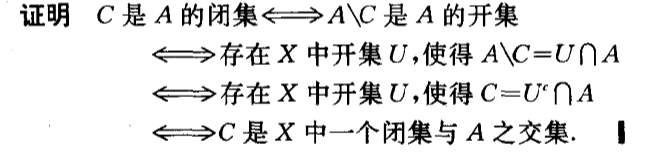既然上面刻画了球形领域，那么邻域怎么能少。说到邻域，内点，聚点，闭包什么的，拓扑空间会不会与数分所学的有所差异？下面将以以介绍。
4.拓扑空间的几个基本概念
既然拓扑是规定了哪些子集是开集，那么下面的概念基本都是以集合语言刻画的。
4.1 邻域、内点与内部
（1）定义：设$A$是拓扑空间$X$的一个子集，点$x \in A$。如果存在开集$U$，使得$x \in U \subset A$，则称$x$是A的一个内点，A是$x$的一个邻域。A的所有内点的集合称为A的内部，记做$A^。$
（2）常用性质
one：若$A \subset B$，则$A^。\subset B^。$;
two：$A^。$是包含在A中的所有开集的并集，因此是包含在A中的最大开集。
three：$A^。=A \iff$A是开集；
four：$(A \bigcap B)^。= A^。\bigcap B^。$
five：$(A \bigcup B)^。\supset A^。\bigcup B^。$
这里只证明two的，其他的较为简单就不证明了。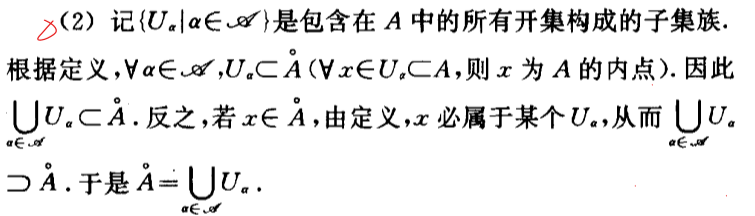4.2 聚点与闭包
啊这，这个是真的很重要，证明老出了，还跟数分上差别较大。
(1)定义：设A是拓扑空间$X$的子集。$x \in X$，如果$x$的每个邻域都含有$A \backslash \lbrace x \rbrace$中的点，则称$x$为A的聚点。A的所有聚点的集合称为A的导集，记做$A'$。称集合$\overline{A}:=A \bigcup A'$为A的闭包。
由定义不难推出：$x \in \overline{A} \iff$$x$的任一邻域与A都有交点。
这里要注意，$x$是$\in X$的，所以它的每个邻域$U$是$\subset X$的而非$\subset A$。
eg： 设$X=\lbrace a,b,c \rbrace$，规定拓扑$\tau = \lbrace X,\varnothing,\lbrace a \rbrace \rbrace$,则当 $A = \lbrace a \rbrace$时，$x=b,c$，其邻域为$U=X$，而$U\backslash x$显然含$a$，即$x=b,c$每个邻域$U \bigcap A\backslash \lbrace x \rbrace \not= \varnothing$，即$x$的每个邻域均含有$A\backslash \lbrace x \rbrace$的点，故$x=b,c$为$A=\lbrace a \rbrace$的聚点。
（2）常用性质：
one：若$A \subset B$，则$\overline{A} \subset \overline{B}$;
two：$\overline{A}$是所有包含A闭集的交集，所以是包含A的最小的闭集；
three：$\overline{A}=A \iff$A是闭集；
four：$\overline{A \bigcup B} = \overline{A} \bigcup \overline{B}$;
five：：$\overline{A \bigcap B} \subset \overline{A} \bigcap \overline{B}$;
这里给出two的证明：设$\lbrace U_{\beta}|\beta\in \lambda \rbrace$是包含A的所有闭集构成的子集族。
4.3 稠密与可分拓扑空间
如果拓扑空间X的子集A，满足$\overline{A}=X$，则称A是稠密的。如果X有可数的稠密子集，则称X是可分拓扑空间。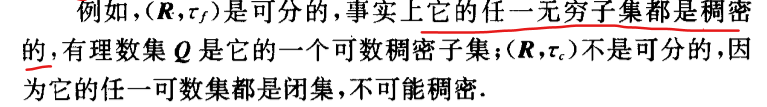$(R,\tau_f$）为余有限拓扑，$(R,\tau_{c})$为余可数拓扑
4.4 序列收敛
这个我们只要了解其定义即可。
设$\lbrace x_n \rbrace$是拓扑空间$X$中点的序列，如果点$x_0 \in X$的任一邻域U都包含$\lbrace x_n \rbrace$的几乎所有项（即只有有限个$x_n$不在U中；或者说存在正整数N，使得当$n > N$时，$x_n \in U$，则说$\lbrace x_n \rbrace$收敛到$x_0$，记做$x_n\rightarrow x_0$。
要注意的是与数分不一样的地方，序列可能收敛到多个点。
例如$(R,\tau_f)$中，只要$\lbrace x_n \rbrace$的项两两不同，则任一点$x \in R$的邻域（有限集的余集）包含$\lbrace x_n \rbrace$的几乎所有项，从而$x_n\rightarrow x$。


展开全文• 稳定性条件取决于紧凑尺寸的长度，并且比具有琐碎拓扑的背景的限制条件要宽松。 真空电流密度是磁通量的周期函数，被紧凑的尺寸所包围，周期等于磁通量量子。 它被明确分解为无边界贡献和边界诱导贡献。 与场平方和...
• 文献3：拓扑空间——高国士 一、写在前面 　现代数学是我目前觉得最美的数学。数学三大分支：代数，几何，分析学。最终毫无例外地用集合论解释了，真是神奇，让人拍案叫绝。以前总是思考，所有的数学到底归根于...
参考：
文献1
文献2
文献3：拓扑空间——高国士
一、写在前面
现代数学是我目前觉得最美的数学。数学三大分支：代数，几何，分析学。最终毫无例外地用集合论解释了，真是神奇，让人拍案叫绝。以前总是思考，所有的数学到底归根于什么？当时学代数，学函数，觉得所有数学就是集合、映射。没想到真有有点不谋而合的感觉。但是没想过分析学用集合论也能解释得毫无破绽。至于几何学，当然学解释几何时，能感觉到有点解方程，用代数解决的感觉。但也没想过用齐次坐标表达，最终任意的几何形式，也能用简洁的式子描述。简言之，集合论让人惊叹不已。
如果没有问数学最底层的理论基础，那么，很多东西变得可笑，如图，你可能证明命题，证来证去都是一个，而自己都不知道，甚至也不知道哪个定理先有，哪个后有的，而把后有的定理证明先有的定理。数学家们致力于把把所有的数学定理建成一套完备的定理，并最终依赖简单的几条公理。看过公理的，你就会发笑，这么简单的东西，还有人去证啊？是的，从最简单的公理出发，树状地推导所有的定理，最终建立一套完备的定理系统。二、简单回顾一下现代数学第一、二章：
1、集合交、并；基、势，映射、可列集、连续集；等价关系、序关系；实数的完备性定理（有限开覆盖定理，公理或许更合适一些，这里用几百个等价命题），其中一个就是极限的定义。
2、二元运算；群、环、模、线性空间；线性算子、线性泛函、张量空间
三、拓扑空间
至此，假设上述知识已经掌握。现在写一下，自己对第三章的拓扑空间的理解。是学习的过程，很可能有许多错误的理解，但是研究不怕出错。
高国士先生的书有400页来解释拓扑空间。我只能略略地叹息。
1、讲拓扑空间，先说用什么用！（工科的心态啊！）。拓扑空间用来自然地从集合概念引入极限的概念，进而为分析学打下基础。另外，拓扑空间可以在等价关系中推导、而不必在序关系中提出极限概念？（待思考）。实际很多概念是忽略这两种关系提出的。
2、先蛮不讲理地提出一些概念：拓扑空间、拓扑基、开集、闭集、邻域、邻域基、Hausdorff space、内点、外点、边界点、孤立点、极限点（累积点）、稠密、有界集、全有界集、列紧集、紧集、收敛、连续映射、同胚映射、连通、度量空间、度量空间的连通性（部分概念只是描述过程中的产物，避免重复写一堆文字才创造出的概念，并无需对它有深刻的理解。这些概念如同代码中的函数，就是避免重复写那段重复代码才创造函数）
3、拓扑空间定义：给定一个集合X，这个集合有许多子集，我们把部分或全部子集收集起来，并要求它们满足一些约束条件，由此组成的空间称为拓扑空间（X，ζ）.满足的约束条件是：任意子集的叫仍在是收集中的某个子集；任意多个子集的并仍是收集中的某个子集。收集中一定有空集和X。
4、拓扑基：在收集的子集中，找到至少数量的子集，通过这些子集本身及一些数量子集之间的并可以创造出收集中的任何一个子集
5、开集、闭集：收集中的任何一个子集都是开集。以X为全集，开集的补集叫做闭集。那么，这里的开集、闭集和以前的开区间、闭区间有没有关系呢？我们也类比一些，假如，开区间类比开集，闭区间类比闭集，什么类比集合X？令某个区间是X，拓扑空间可能无数个X的子区间的组成的族，闭区间就是X-这个开区间。我们引用文献2中的一个解释：
Closed set（闭集合）。在现代的拓扑学的公理化体系中，开集和闭集是最基本的概念。一切从此引申。这两个概念是开区间和闭区间的推广，它们的根本地位，并不是一 开始就被认识到的。经过相当长的时间，人们才认识到：开集的概念是连续性的基础，而闭集对极限运算封闭——而极限正是分析的根基。
很明显，这不能说明离散拓扑的意义？在我看来，闭区间和开区间是特殊的闭集和开集。我们看下图。线段代表X（无论开集或闭集），中间中括号代表开集，两侧代表闭集。我们发现两侧也属于X，也算开集。这提示我们，这里举的例子很特殊，及开区间、闭区间也是特殊的开集、闭集。我们下面可以看出这点。6、还是先提一下：平凡拓扑（最粗）、离散拓扑（最细）：所有子集构成、通常拓扑：数直线上的开集族连通空间及R形成R上的通常拓扑。摘：在离散拓扑空间中，任何子集都是闭集，也同时是闭集，任何子集的闭包就是自己。平凡空间非空子集的闭包都是X。P22
7、设X的子集U包含着某个开集，该开集包含着x，称U为x的邻域；如果U是开集，则称U是x的开邻域。
8、Hausdorff space空间。（T2空间）
因为一般的拓扑空间研究比较单一、抽象，我们会逐渐给出一些约束条件。
首先：T0空间。其次：T1空间。接着：T2空间。再者，T3：最后，T4：转载于:https://www.cnblogs.com/Wanggcong/p/4922688.html
展开全文• 1、引言 作者：qang pan ... 来源：知乎 著作权归作者所有。... 什么是赋范线性空间、内积空间，度量空间，希尔伯特空间 ？ 现代数学的一个特点就是以集合为研究对象，这样的好处就是可以将很多
1、引言
什么是赋范线性空间、内积空间，度量空间，希尔伯特空间 ？
现代数学的一个特点就是以集合为研究对象，这样的好处就是可以将很多不同问题的本质抽象出来，变成同一个问题。
函数空间/ 集合，最重要的是，1.元素；2、规则。
例如，线性空间，定义的元素：向量；规则：8大规则
但线性空间（线性代数课学的）中的元素没有“长度”（相当于三维空间中线段的长度），为了量化线性空间中的元素，所以又在线性空间引入特殊的“长度”，即范数。赋予了范数的线性空间即称为赋笵线性空间。
但赋范线性空间中两个元素之间没有角度的概念，为了解决该问题，所以在赋范线性空间中又引入了内积的概念。
因为有度量，所以可以在度量空间、赋范线性空间以及内积空间中引入极限，但抽象空间中的极限与实数上的极限有一个很大的不同就是，极限点可能不在原来给定的集合中，所以又引入了完备的概念，完备的内积空间就称为Hilbert空间。
这几个空间之间的关系是：线性空间与度量空间是两个不同的概念，没有交集。赋范线性空间就是赋予了范数的线性空间，也是度量空间（具有线性结构的度量空间，内积空间是赋范线性空间，希尔伯特空间就是完备的内积空间。
2、完备空间
完备空间或者完备度量空间是具有下述性质的空间：空间中的任何柯西序列都收敛在该空间之内。
任一紧致度量空间都是完备的。
实际上，一个度量空间是紧致的当且仅当该空间是完备且完全有界的。
完备空间的任一子空间是完备的, 当且仅当它是一个闭子集。
若X为一集合，M是一个完备度量空间，则所有从X映射到M的有界函数f的集合B(X, M)是一个完备度量空间，其中集合B(X, M)中的距离定义为：d(f,g):=sup⁡{ d(f(x),g(x)):x∈X }d(f,g):=sup⁡{ d(f(x),g(x)):x∈X }{\displaystyle d(f,g):=\sup \left\{\,d(f(x),g(x)):x\in X\,\right\}} d(f,g) := \sup\left\{\,d(f(x),g(x)) : x\in X \,\right\}。
若X为一拓扑空间，M是一个完备度量空间，则所有从X映射到M的连续有界函数f的集合Cb(X,M)是B(X, M)（按上一条目的定义）中的闭子集，因而也是完备的。
贝尔纲定理：任一完备度量空间为一贝尔空间。就是说，该空间的可数个无处稠密子集的并集无内点。
3、希尔伯特空间
在数学里，希尔伯特空间即完备的内积空间，也就是说一个带有内积的完备向量空间。是有限维欧几里得空间的一个推广，使之不局限于实数的情形和有限的维数，但又不失完备性（而不像一般的非欧几里得空间那样破坏了完备性）。
此外，希尔伯特空间还是一个完备的空间，其上所有的柯西序列会收敛到此空间里的一点，从而微积分中的大部分概念都可以无障碍地推广到希尔伯特空间中。
希尔伯特空间为基于任意正交系上的多项式表示的傅立叶级数和傅立叶变换提供了一种有效的表述方式，而这也是泛函分析的核心概念之一。希尔伯特空间是公设化数学和量子力学的关键性概念之一。
在希尔伯特空间，所有元素运算取极限，仍然在这个空间内（或者定义的集合内）
4、拓扑空间
减少对距离的定义，提出了连续概念。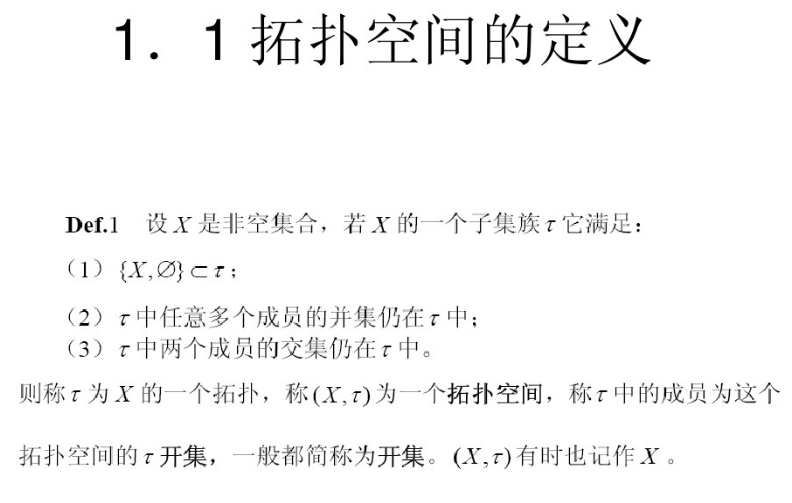X的最简单的拓扑τ={X，∅}，这个是平凡拓扑；
包含X所有子集的拓扑为，离散拓扑。
X是元素，τ是拓扑结构，组成一个拓扑空间。
最近开通了个公众号，主要分享python学习相关内容，推荐系统，风控等算法相关的内容，感兴趣的伙伴可以关注下。公众号相关的学习资料会上传到QQ群596506387，欢迎关注。
参考：

网易云公开课 函数空间;

wikipedia 完备空间;

wikipedia 希尔伯特空间;

wikipedia 拓扑空间;

zhihu 作者：qang pan；

百度文库 拓扑空间；

百度文库 拓扑空间 2;


展开全文• 原帖太多LaTeX，悲剧了，不能直接复制过来了，只好重新整理一遍。  ↑正文前先来一个专业的分割线↑ 所谓拓扑，是一种数学结构。 对于一个集合 E，如果 E 的...就称 T 为 E 上的一个拓扑（Topology）

原帖太多LaTeX，悲剧了，不能直接复制过来了，只好重新整理一遍。

↑正文前先来一个专业的分割线↑

所谓拓扑，是一种数学结构。

对于一个集合 E，如果 E 的一个子集族 T 满足：

空集和全集 E 属于 T；
T 中任意元素的并还是属于 T；
T 中任意有限个元素的交还是属于 T。

就称 T 为 E 上的一个拓扑（Topology） 。

称 (E,T) 为拓扑空间（Topological Space）。

任一集合 E 至少存在两个拓扑：

平凡拓扑：只有空集和全集 E 两个元素的子集族；离散拓扑：E 的所有子集组成的子集族。

开集（open set）在拓扑学中定义比在分析学中要宽。分析中，开集是指点都是内点的集合；拓扑学中，开集和拓扑是紧密相连的，它指的是拓扑的成员，具体由特定的拓扑决定。拓扑学的定义是蕴含分析学的定义的。

另外，闭集（closed set）可以视为补集是开集的集合。

点 x 的邻域（neighborhood）定义是：

存在一个包含点 x 的开集 V ，使得点集 U 包含开集 V，则称 U 为 x 的一个邻域。

拓扑学中的收敛（converge），是指对于点列 {x_n}，和某点 x 与它的任意邻域 U(x)，存在某个正整数 N，在n大于N之后，点列中所有的元素都属于邻域 U(x) ——就可以说点列 {x_n} 收敛到 x 了。

看上去和分析学中的收敛定义一样？哼哼，注意这可是拓扑空间哦。

在拓扑空间里，点列的收敛点有时候不止一个，可以是两个、三个、甚至多个。这也是拓扑空间反直观的地方。我总认为，真要在脑海中中给拓扑空间一个直观的图像，是不大可能的；但某些特定的拓扑空间的图像，可以有助学习理解——譬如分析学中的 n 维欧氏空间，也可以是拓扑空间的特例；拓扑学中的所有结论，在分析学中都可成立。

还有拓扑空间的分割，对应有一类特殊的空间：T1、T2、T3、T4。

只说明一下T2，它也称豪斯多夫空间（Hausdorff space），其定义是在空间中的每任意两点，都能找到各自的不相交邻域。

在豪斯多夫空间，收敛点就是唯一的了。

因为欧氏空间也是豪斯多夫空间的一种，对应到欧氏空间，会更好理解什么是「邻域不交」。如图所示↓再来一个专业的分割线↓

这门泛函MOOC酷毙了，开始上课后连点集拓扑和实变函数也顺带学了，一次性满足三个愿望啊！

展开全文• 拓扑空间： 给定集x,它的一个子集族J称为x上的一个拓扑结构,简称拓扑,是指J满足下列三个条件：①空集和x本身是J的元;②J内任意有限多个元的交仍是J的元；③J内任意多个元的并仍是J的元。集x连同它上面的一个拓扑J,...数据结构
• 在这项研究中，我们分析了具有非平凡拓扑的时空中标量粒子波动方程的解。 考虑该时空的两种配置，找到了Klein-Gordon振荡器的解决方案。 在第一个空间中，假定S1×R3空间，其中将度量写在通常的惯性参考系中。 在第...
• 在这项工作中，我们研究了在重力场与背景曲率存在非最小耦合的情况下，一... 我们通过在拓扑上琐碎的平面Gödel型度量中分析平面和弯曲空间中的Dirac方程，来获得该相对论系统的允许能量，并分析其对能量特征值的影响。
• 学GIS空间数据库的时候，拓扑方面内容笔记 拓扑的定义 拓扑是研究几何图形或空间在连续改变形状后还能保持不变的一些性质的一个学科。它只考虑物体间的位置关系而不考虑它们的形状和大小。 “拓扑”就是把实体...webgis
• 基础范畴论 1. 范畴：一个范畴C，包括对象类Ob(C)，态射类，表示Ob(C)中的所有对象间的态射构成的类，满足 （1）态射复合性：是复合，记作，简记为fg ...Top：拓扑空间与连续映射； Man：拓...
• ## 拓扑--点集拓扑

千次阅读 2020-12-21 12:02:38
它与良序定理等价 拓扑空间 （1）拓扑空间（拓扑结构）：集合X上的一个子集族，满足 包含在中（平凡性）；中的任意多成员并在中 （任意并）；中的有限多成员的交在中 （有限交），则称子集族是X的一个拓扑，X和一起...
• （1）几何独立（仿射独立）：N维欧氏空间中的一个点集称为几何独立或仿射独立的，如果等式 仅在每一个纯量时才成立。这等价于中的向量集线性独立 （2）n-维平面：设 为中的一个几何独立点集，由它确定的点x的...
• p维相对上同调群： 上链映射：单纯映射 诱导链映射 ，它的对偶 称为上链映射，其诱导上同调群的同态为 （8）奇异上同调群：(X, A) 为拓扑空间偶，A为X的子空间，G是一个Abel群 p维奇异上链群： ，它是自由...
• 拓扑研究的是图形变化中的不变。橡皮几何学。 如果图形MMM能变成图形M′M'M′，就称为一个拓扑变换，二者是同胚的，拓扑性质就是同胚的图形所共有的几何性质。说明拓扑性质正是变中的不变。 ...
• 我们描述了由Dai和Freed引起的关于Atiyah-Patodi-Singer eta不变量的定理的物理推导，这对于物质的异常和拓扑阶段很重要。... 基态取行列式束中的值，并且具有不平凡的Berry相，该相代表了低能拓扑相的特征。
• 拓扑学是数学的一个分支，研究物体在连续变形下的性质不变性。数学之外的其他领域也在研究拓补结构。当引入拓扑结构时，通常会想到一个著名的例子：一个甜甜圈(X)和一个杯子(Y)是两个拓扑上相同的物体，它们都是环面...
• 1. 平凡(trivial)就是指最简单的...拓扑空间平凡子空间都是全空间本身和空集，这两个子空间是很容易想到的子空间，但是对具体问题一般没什么用或者很容易讨论，通常更关心非平凡的子空间的情况。 3.关于平凡，...flex 人工智能 动态规划 算法 机器学习
• Lovelock重力理论为研究高曲率项在AdS / CFT中... 这些解一般化了准拓扑重力的黑洞解，并且存在于与因果关系和其他一致性条件引起的约束一致的参数空间区域中。 它们具有有限的保守电荷，并表现出非平凡的热力学性质。
• 前一节传送: 分离性(下)本文旨在整理Sorgenfrey直线 的拓扑性质, 主要考虑可数性和分离性, 并附上简要的证明. 它的性质在上有一个不错的综述. 题图来源于. 目录(1). 简单性质(2). 可数性(3). 分离性(4). ...
• 我们考虑具有两个Dirac节点的拓扑Dirac半金属的流体力学模型，这些节点在动量空间中沿旋转轴分开。 有人认为，除手性异常外，所讨论的系统还具有另一个ℤ2。 为了对这样的系统进行建模，我们引入了两个U（1）规场。 ...
• 以下内容全部摘自维基百科或教科书。 为了后续查阅方便，故此记录。 1. 集合 1.1 σ代数 某个集合 X 上的σ-代数又叫 σ-域，是 X 的幂集的子集合（X 的幂集即包含所有 X 的...XXX是一个集合，XXX上的拓扑（topograp
• 拓扑学——探寻大数据的内在模式 点击上方“大数据文摘”可以订阅哦！ 大数据文摘作品，欢迎个人转发朋友圈；其他机构、自媒体转载，务必后台留言，申请授权。 作者：Kevin Knudson编译：数码叮叮 校对：于丽君...
• fprintf('sol中每个拓扑之间以8为界，其中包含所有X上非平凡拓扑') 运行成功，numm=27，共27个非平凡拓扑。 下面将matlab语言翻译为python语言  交运算： def intersection(x): import numpy x=...
• 首先说说空间(space)，这个概念是现代数学的命根子之一，从拓扑空间开始，一步步往上加定义，可以形成很多空间。线形空间其实还是比较初级的，如果在里面定义了范数，就成了赋范线性空间。赋范线性空间满足完备性，......